Next: Derivation of lunar equations Up: Lunar motion Previous: Earth-Moon-Sun system

# Solar orbit

Let us set up a conventional Cartesian coordinate system which is such that the (apparent) orbit of the Sun about the Earth-Moon barycenter lies in the-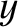plane. This implies that the-plane corresponds to the so-called ecliptic plane. Approximating the solar orbit as a low-eccentricity Keplerian orbit, we can write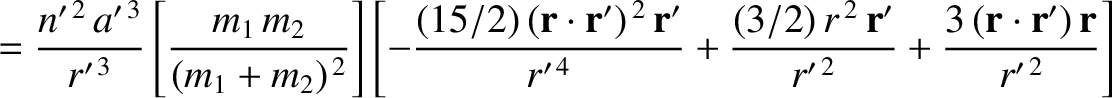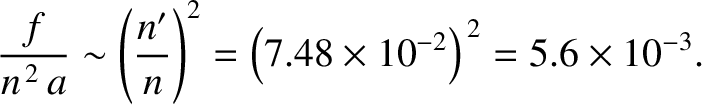(11.41)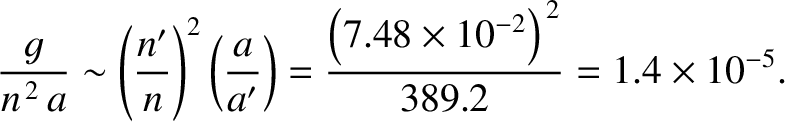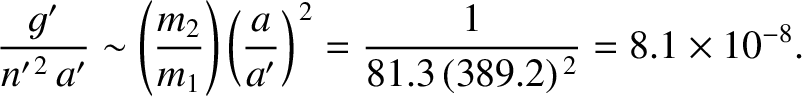(11.42) and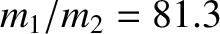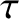(11.43)

where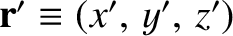, and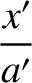is the orbital eccentricity (Yoder 1995). (See Section 4.13.) Here, we have neglected terms that are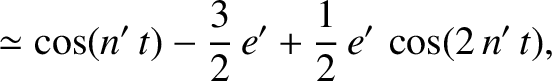, and smaller.

Richard Fitzpatrick 2016-03-31